# Molar Mass of Gold - Gold Nanoparticle Properties - Molecular Weight of 1 Gold Nanoparticle

### Important reference for determining gold nanoparticles molecular weight, surface area, volume, and weight of one nanoparticle and defining the molar mass of gold and density of gold

The molar mass of gold = 196.96657 g/m. The molar mass of gold is defined as the mass of 1 mole of gold atoms - typically specified in grams. Therefore the molar mass of gold is stated in units of grams/mole or g/m. It is also frequently called the Molecular Weight of Gold.

The Molecular Weight of gold nanoparticles is slightly different. It is defined as the weight of one mole of gold nanoparticles (not atoms). This value changes of course with the size of the gold nanoparticles.

The density of gold = 19.3 g/cm3. The density of gold is defined as the mass of gold atoms found in a specific volume. Typically it is stated in units of grams/cubic centimeter or g/cm3. For calculating in nanoparticle sizes, that number becomes 19.3 x 10(-21) g/nm3.

The following shows the equations to calculate the Surface Area, Volume, and Weight of one gold nanoparticle, as well as calculating the Molecular Weight in kDa of a mole of gold nanoparticles.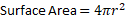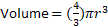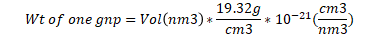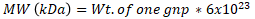The following shows the equations to calculate the Surface Area, Volume, and Weight of one gold nanorod, microgold, or gold nanowire, as well as calculating the Molecular Weight in kDa of a mole of gold nanorods, microgold, or gold nanowires.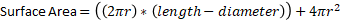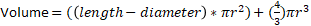The following interactive table calculates the molecular weight, as well as the surface area, volume, and weight of one nanoparticle. This will work for spherical gold nanoparticles, gold nanorods, microgold, and gold nanowires.